Paper Analysis: SSC CGL General Intelligence & Reasoning (Pr - Study24x7Default error msg

New to Study24x7 ?
By continuing, you agree to Study24x7 ?

# Paper Analysis: SSC CGL General Intelligence & Reasoning (Previous Year Question Paper 28th August 2016) Answer Key

Updated on 08 April 2021SSC Preparation Strategies & S
Updated on 08 April 2021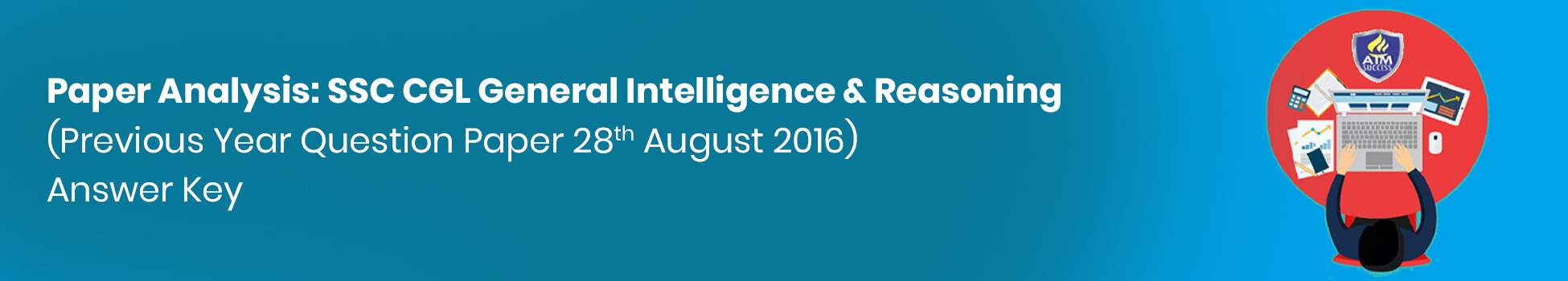In this article, I am providing the solution with the supporting explanation of the previous Year SSC CGL question Paper of General Intelligence & Reasoning for the help of SSC CGL aspirants working hard on their preparation. As the General Intelligence & Reasoning is one of the most scoring sections in the SSC CGL syllabus. The aspirants are required to practice as many questions to boost their scores.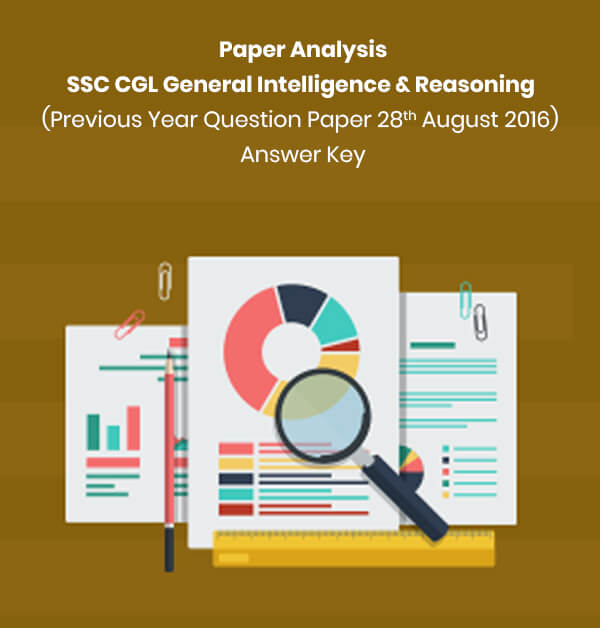1. Select the related word/letters/numbers from the given alternatives:

Electric Current : Ampere :: Weight : ?

a.Scale

b.Kilogram

c.Commodity

d.Measurement

Explanation: Option (b) i.e. from all the given alternatives Kilogram is the right answer. As electric current is measured in ‘ampere’ the weight is measured in ‘kilogram’.

2. Select the related word/letters/numbers from the given alternatives:

ACXZ : BDWY :: CEVX : ?

a.DFUZ

b.DFUW

c.DGUW

d.DFUV

Explanation: Option (b) i.e. the set of alphabets ‘DFUW’ is the right answer. In the given analogy, A is replaced by B, C is replaced by D, X is replaced by W and Z with Y. Hence the rule of substitution will be (+1)( +1)( -1)(-1).

3. Select the related word/letters/numbers from the given alternatives:

37 : 65 :: 50 : ?

a.75

b.79

c.82

d.87

Explanation: Option (c) i.e. the number 82 is the right answer.

As 37= (6)2+1, 65= (6+2)2+1. So the analogy is (n+2)2 + 1 if 50= (7)2+1, then 82= (7+2)2+1= (9)2+1.

4. Find the odd word/letters/number from the given alternatives.

a.Thermometer

b.Barometer

c.Diameter

d.Hygrometer

Explanation: Option (c) i.e. the Diameter is the right answer from given alternatives. Here all three except ‘Diameter’ are the instrument used to measure different physical quantities like temperature, atmospheric pressure, and humidity respectively.

5. Find the odd word/letters/number from the given alternatives.

a.JF

b.UQ

c.PL

d.XS

Explanation: Option (d) is the right answer. Here on each pair of alphabet, the applicable rule is the second alphabet= (First alphabet- 4 positions) except ‘XS’.

6. Find the odd word/letters/number from the given alternatives.

a.46-52

b.56-62

c.66-72

d.76-84

Explanation: Option (d) i.e. 76-84 is the right answer. Here on each pair of numbers, the difference of digits is 6 whereas in 76-84 the difference of digits is 8.

7. Arrange the following words as per their order in the dictionary

1. Embody
2. Embrace
3. Embitter
4. Emblem
5. Embroidery

a.3, 4, 2, 5, 1

b.4, 2, 5, 1, 3

c.4, 1, 2, 5, 3

d.3, 4, 1, 2, 5

Explanation: Option (d) i.e. the order 3, 4, 1, 2, 5 is the right answer. If we arrange the given words in the alphabetical order they appear in the dictionary we get

Embitter

Emblem

Embody

Embrace

Embroidery

8. A series is given, with one term missing. Choose the correct alternative from the given ones that will complete the series.

CBA, FED, IHG, LKJ, ?

a.PRT

b.VUT

c.ONM

d.ZYX

Explanation: Option (c) i.e. ‘ONM’ is the correct alternative to complete the series. Here all sets of alphabets in the question are in the reverse order of their occurrence the same as ONM.

ABC

DEF

GHI

JKL

MNO

9. A series is given, with one term missing. Choose the correct alternative from the given ones that will complete the series.

6, 12, 36, 144, 720, 4320, ?

a.30420

b.30240

c.31240

d.32240

Explanation: Option (b) is the right answer. The applied rule here the applicable rule is:

Next number= Previous number* (2,3…n).

6*2=12

12*3=36

36*4= 144

144*5=720

720*6=4320

4320*7= 30240

10. Introducing a woman, a man said "Her mother is the only daughter of my mother-in-law". How is the man related to the woman?

a.Son

b.Brother

c.Husband

d.Father

Explanation: Option (d) i.e. Man is the ‘father’ of the woman in this context. Here in the relationship, he is referring to the relation in terms of her wife.

11.Five children are standing in a row. O is third from M who is standing left of N, and P is on the left of Q who is second from N. What is the position of P from right?

a.Third

b.First

c.Fourth

d.Second

Explanation: Option (d) i.e. ‘P’ is at the second position from right. The right order in which children are standing in the row will be:

N-M-Q-P-O

12.From the given alternative words, select the word which cannot be formed using the letters of the given word:

INTELLIGENCE

a.GENTLE

b.TEEN

c.GREEN

d.ENGINE

Explanation: Option (c) i.e. word ‘GREEN’ is the right answer.

The word ‘INTELLIGENCE’ is not having alphabet ‘R’ in it thus ‘GREEN’ cannot be formed from the letters of given word.

13. If "GOAT" is coded as "HPBU", then how will "FROG" be coded as?

a.GSPH

b.PHSG

c.GSHP

d.PSHG

Explanation: Option (a) i.e. “GSPH” is the right answer. Here the coding rule will be (Alphabet+1).

By applying the rule on “GOAT”, you will get “HPBU”

G+1= H

O+1=P

A+1=B

T+1= U

Now apply the coding rule on “FROG”, you will get

F+1=G

R+1=S

O+1=P

G+1= H

14.In a certain code language, '@' represents '+' , '⊕' represents '-' , 'α' represents '÷' and 'θ' represents 'x' . Find out the answer to the following question:

8900α100⊕5θ4⊕121α11 = ?

a.58

b.62

c.158

d.205

Explanation: Option (a) i.e. 58 is the right answer.

When we apply the coding rule of symbols, we get the following expression:

8900÷100-5x4-121÷11

By applying BODMAS rule on above arithmetic expression, we get

89-5x4-11

89-20-11

58

15. Some equations are solved on the basis of a certain system. On the same basis, find out the correct answer from amongst the four alternatives to the unsolved equation

1 × 2 × 3 = 231

3 × 4 × 5 = 453

5 × 6 × 7 = ?

a.657

b.675

c.756

d.765

Explanation: Option (b) i.e. 675 is the right answer. From the given system we can find the pattern arrangement as:

Second digit Third digit First digit

On the basis of the above system, we get 5x6x7= 675

16. Select the missing numbers from the given alternatives-

a.12

b.18

c.20

d.87

Explanation: Option (d) i.e. 87 is the right answer. As the equation which satisfies the given numbers is 1st element+ second element- 8= x, let the x is the missing number in the question.

17. Rajneesh started walking towards North. He took a left turn after walking 20 metres. He again took a right turn after walking 30 metres. Which direction is he facing now?

a.North

b.East

c.West

d.South

Explanation: Option (a) i.e. he is facing north direction. After starting from the north direction when the person will move to the left and then to its right, he will be in the same direction from where he started walking and thus the ‘north’ direction is the right answer.

18. Consider the given statement/s to be true and decide which of the given conclusions/assumptions can definitely be drawn from the given statement.

Statements:

All books are trees.

All trees are lions.

Conclusions:

All books are lions.

All lions are books.

All trees are books

Some lions are books.

a.Only conclusions I and III follow

b.Only conclusions I and IV follow

c.None of the conclusions follow

d.All conclusions follow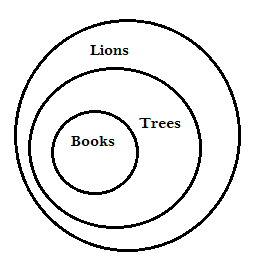Explanation: Option (b) i.e. only conclusion I and conclusion IV will be drawn from the given statement. From the given statement, we can deduce that ‘All books are lions’ and ‘Some lions are books’.

19. In the given cube, which color is opposite to Blue?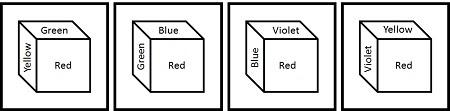a.Green

b.Red

c.Violet

d.Yellow

Explanation: Option (d) i.e. yellow is the color that is opposite to Blue in the cube. As the direction in which the colors are assigned to the cube in the anti-clockwise direction and thus the color yellow is the right answer.

20. Identify the relationship that best represents the relationship among classes given below:

Professors, Women and Clerks

1)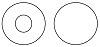2)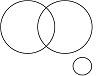3)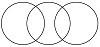4)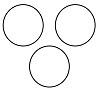Explanation: Option (3) is representing the best relation among the classes of professors, women and, clerks. In the context, a woman may be a professor as well as a clerk which is not evident in any other relationship than (3).

21.Which answer figure will complete the pattern in the figure?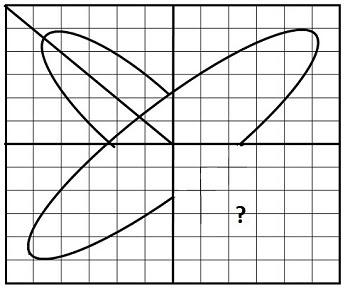a)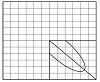b)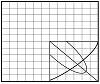c)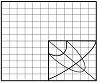d.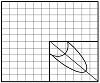Explanation: The figure provided in the option (d) will complete the above question figure. In the question, the problem is on the mirror-image and the figure in option (d) is the mirror image of the pattern shown in the IInd quadrant.

22. From the given answer figures, select the one in which the figure is hidden/embedded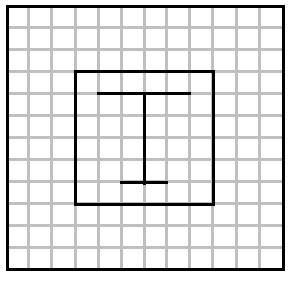a.b.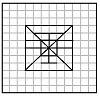c.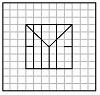d.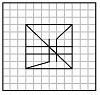Explanation: The figure provided in the option (b) is embedded in the question figure. Thus option (b) will be the right answer. The problem in the question can be solved by superimposing the given figure on each of the answer options.

23.A piece of paper is folded and cut as shown below in the figures. From the given answer figures, indicate how it will appear when opened.a.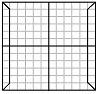b.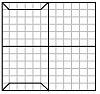c.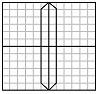d.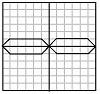Explanation: The figure provided in the answer option (d) is the right answer. You need to visualize the problem in the question on a folding paper with the same structure and will get the figure as given in the option (d).

24.If a mirror is placed on the line MN, then which of the answer figures is the right image of the given figure?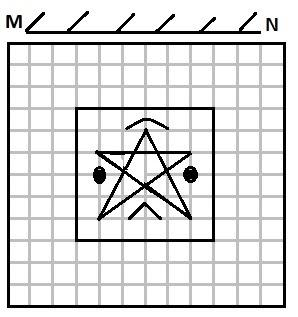a.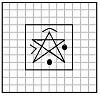b.c.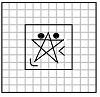d.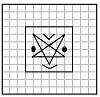Explanation: The figure given in the answer option (d) is the right mirror image of the question figure. In the given problem, you will get the answer figure if you rotate the figure by 1800 in the clockwise direction along with the mirror.

25. A word is represented by only one set of numbers as given in any one of the alternatives. The sets of numbers given in the alternatives are represented by two classes of alphabets as in two matrices given below. The columns and rows of Matrix I are numbered from 0 to 4 and that of Matrix II are numbered from 5 to 9. A letter from these matrices can be represented first by its row and next by its column, e.g., 'D' can be represented by 01, 13, etc., and 'H' can be represented by 55, 67, etc. Similarly you have to identify the set for the word 'LIKE'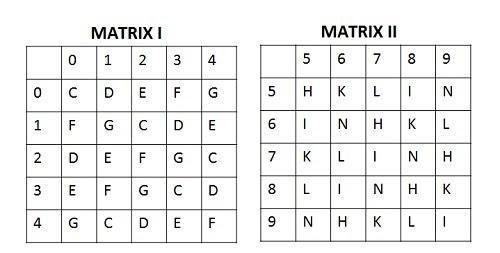a.56, 58, 03, 02

b.57, 65, 62, 02

c.56, 62, 03, 02

d.57, 68, 40, 02

Explanation: Option (b) i.e. the arrangement of alphabet as the set 57, 65, 62, 02 is the right representation of “LIKE”.

Representation code for L: 57, 69, 76, 85, 98

Representation code for I: 58, 65, 77, 86, 99

Representation code for K: 56, 68, 75, 89, 97

Representation code for E: 02, 14, 21, 30, 43

So we can find the best possible set from the complete representation set and we get the answer as 57, 65, 62, 02.

Write a comment...Trending Articles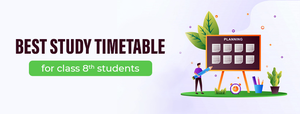By CBSE CLASS 8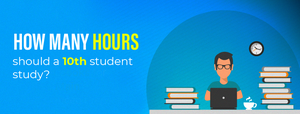By CBSE CLASS 10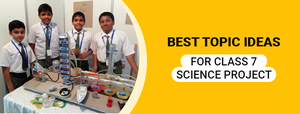By CBSE CLASS 7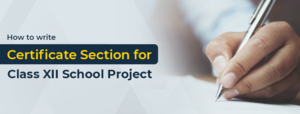By Edubabble LLP
Related PostsServicesNeed Some Help!Connect